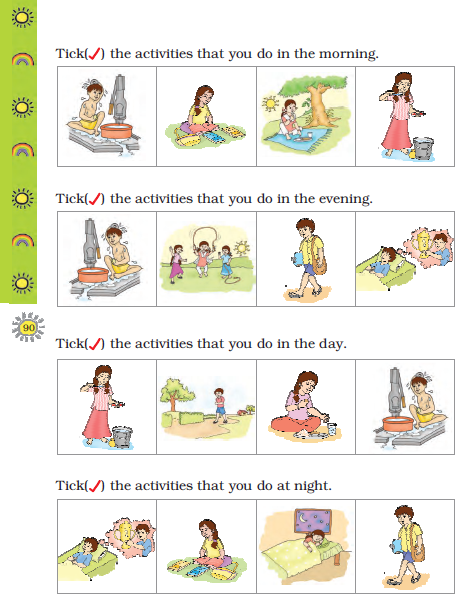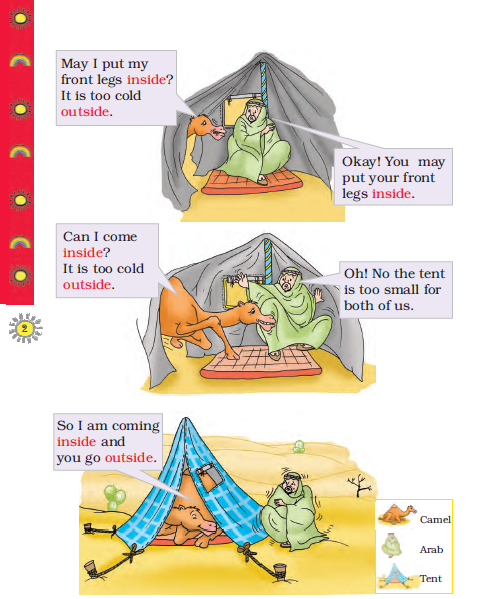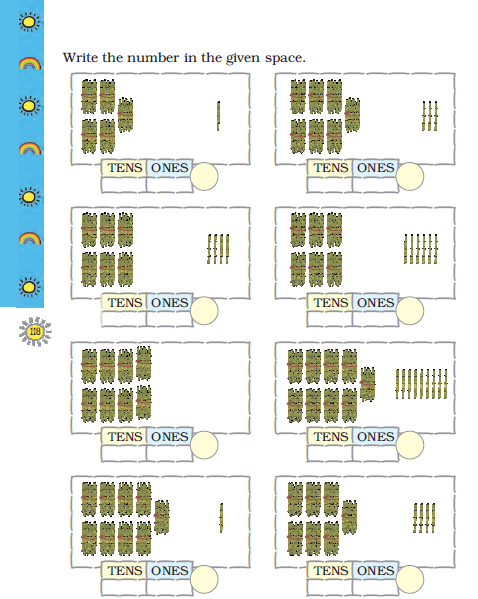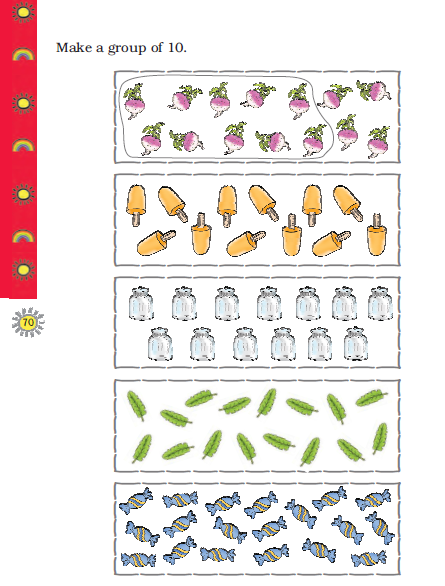NCERT Class 1 Mathematics

• NCERT Class 1 Maths Time

Please refer to attached file for NCERT Class 1 Maths - Time

• NCERT Class 1 Maths Subtraction

Please refer to attached file for NCERT Class 1 Maths - Subtraction

• NCERT Class 1 Maths Shapes and Space

Please refer to attached file for NCERT Class 1 Maths - Shapes and Space

• NCERT Class 1 Maths Patterns

Please refer to attached file for NCERT Class 1 Maths - Patterns

• NCERT Class 1 Maths Numbers

Please refer to attached file for NCERT Class 1 Maths - Numbers

NCERT Class 1 Maths TimePlease refer to attached file for NCERT Class 1 Maths - Time

Click for more Mathematics Study Material
 NCERT Class 1 Maths Patterns NCERT Class 1 Maths Measurement NCERT Class 1 Maths Shapes and Space NCERT Class 1 Maths Money NCERT Class 1 Maths Subtraction NCERT Class 1 Maths Numbers from One to Nine NCERT Class 1 Maths Time NCERT Class 1 Maths Numbers from Ten to Twenty NCERT Class 1 Maths Addition NCERT Class 1 Maths Numbers from Twenty one to Fifty NCERT Class 1 Maths Data Handling NCERT Class 1 Maths Numbers NCERT Class 1 Maths How Many

NCERT Class 1 Maths SubtractionPlease refer to attached file for NCERT Class 1 Maths - Subtraction

Click for more Mathematics Study Material
 NCERT Class 1 Maths Patterns NCERT Class 1 Maths Measurement NCERT Class 1 Maths Shapes and Space NCERT Class 1 Maths Money NCERT Class 1 Maths Subtraction NCERT Class 1 Maths Numbers from One to Nine NCERT Class 1 Maths Time NCERT Class 1 Maths Numbers from Ten to Twenty NCERT Class 1 Maths Addition NCERT Class 1 Maths Numbers from Twenty one to Fifty NCERT Class 1 Maths Data Handling NCERT Class 1 Maths Numbers NCERT Class 1 Maths How Many

NCERT Class 1 Maths Shapes and SpacePlease refer to attached file for NCERT Class 1 Maths - Shapes and Space

Click for more Mathematics Study Material
 NCERT Class 1 Maths Patterns NCERT Class 1 Maths Measurement NCERT Class 1 Maths Shapes and Space NCERT Class 1 Maths Money NCERT Class 1 Maths Subtraction NCERT Class 1 Maths Numbers from One to Nine NCERT Class 1 Maths Time NCERT Class 1 Maths Numbers from Ten to Twenty NCERT Class 1 Maths Addition NCERT Class 1 Maths Numbers from Twenty one to Fifty NCERT Class 1 Maths Data Handling NCERT Class 1 Maths Numbers NCERT Class 1 Maths How Many

NCERT Class 1 Maths PatternsPlease refer to attached file for NCERT Class 1 Maths - Patterns

Click for more Mathematics Study Material
 NCERT Class 1 Maths Patterns NCERT Class 1 Maths Measurement NCERT Class 1 Maths Shapes and Space NCERT Class 1 Maths Money NCERT Class 1 Maths Subtraction NCERT Class 1 Maths Numbers from One to Nine NCERT Class 1 Maths Time NCERT Class 1 Maths Numbers from Ten to Twenty NCERT Class 1 Maths Addition NCERT Class 1 Maths Numbers from Twenty one to Fifty NCERT Class 1 Maths Data Handling NCERT Class 1 Maths Numbers NCERT Class 1 Maths How Many

NCERT Class 1 Maths NumbersPlease refer to attached file for NCERT Class 1 Maths - Numbers

Click for more Mathematics Study Material
 NCERT Class 1 Maths Patterns NCERT Class 1 Maths Measurement NCERT Class 1 Maths Shapes and Space NCERT Class 1 Maths Money NCERT Class 1 Maths Subtraction NCERT Class 1 Maths Numbers from One to Nine NCERT Class 1 Maths Time NCERT Class 1 Maths Numbers from Ten to Twenty NCERT Class 1 Maths Addition NCERT Class 1 Maths Numbers from Twenty one to Fifty NCERT Class 1 Maths Data Handling NCERT Class 1 Maths Numbers NCERT Class 1 Maths How Many

NCERT Class 1 Maths Numbers from Twenty one to FiftyPlease refer to attached file for NCERT Class 1 Maths - Numbers from Twenty-one to Fifty

Click for more Mathematics Study Material
 NCERT Class 1 Maths Patterns NCERT Class 1 Maths Measurement NCERT Class 1 Maths Shapes and Space NCERT Class 1 Maths Money NCERT Class 1 Maths Subtraction NCERT Class 1 Maths Numbers from One to Nine NCERT Class 1 Maths Time NCERT Class 1 Maths Numbers from Ten to Twenty NCERT Class 1 Maths Addition NCERT Class 1 Maths Numbers from Twenty one to Fifty NCERT Class 1 Maths Data Handling NCERT Class 1 Maths Numbers NCERT Class 1 Maths How Many

NCERT Class 1 Maths Numbers from Ten to TwentyPlease refer to attached file for NCERT Class 1 Maths - Numbers from Ten to Twenty

Click for more Mathematics Study Material
 NCERT Class 1 Maths Patterns NCERT Class 1 Maths Measurement NCERT Class 1 Maths Shapes and Space NCERT Class 1 Maths Money NCERT Class 1 Maths Subtraction NCERT Class 1 Maths Numbers from One to Nine NCERT Class 1 Maths Time NCERT Class 1 Maths Numbers from Ten to Twenty NCERT Class 1 Maths Addition NCERT Class 1 Maths Numbers from Twenty one to Fifty NCERT Class 1 Maths Data Handling NCERT Class 1 Maths Numbers NCERT Class 1 Maths How Many

NCERT Class 1 Maths Numbers from One to NinePlease refer to attached file for NCERT Class 1 Maths - Numbers from One to Nine

Click for more Mathematics Study Material
 NCERT Class 1 Maths Patterns NCERT Class 1 Maths Measurement NCERT Class 1 Maths Shapes and Space NCERT Class 1 Maths Money NCERT Class 1 Maths Subtraction NCERT Class 1 Maths Numbers from One to Nine NCERT Class 1 Maths Time NCERT Class 1 Maths Numbers from Ten to Twenty NCERT Class 1 Maths Addition NCERT Class 1 Maths Numbers from Twenty one to Fifty NCERT Class 1 Maths Data Handling NCERT Class 1 Maths Numbers NCERT Class 1 Maths How Many

Latest NCERT & CBSE News

Read the latest news and announcements from NCERT and CBSE below. Important updates relating to your studies which will help you to keep yourself updated with latest happenings in school level education. Keep yourself updated with all latest news and also read articles from teachers which will help you to improve your studies, increase motivation level and promote faster learning

Surya Namaskar Project on 75th Anniversary of Independence Day

Ministry of Education, Govt of India vide letter No. F.No. 12-5/2020-IS-4 dated 16.12.2021 has intimated that under the banner Azadi ka Amrit Mahotsav the National Yogasanasports Federation has decided to run a project of 750 million Surya Namaskar from 01 January 2022...

This is with reference to CBSE Notification No. 40/2021 dated 04.05.2021 regarding the Innovation Ambassador program – An online training program for teachers by CBSE in collaboration with Ministry of Education’s Innovation Cell (MIC) and AICTE. In view of the current...

Pariksha Pe Charcha 2022

The 5th edition of Pariskhas Pe Charcha the unique interactive program of Hon’ble Prime Minister with students teaches and parents will be held through virtual mode in February, 2022. In order to select participants who will be featured in Pariksha Pe Charcha programme...

Score well in Class 12 English Boards Exam

12th Board exams are an important part of students' lives. The marks obtained in the board exam decide the college in which one can study. In class 12 the syllabus of each and every subject increases vastly and it is difficult to cover up every point. In English also...

MCQ Question based CBSE examination

For 2021-22 CBSE has launched MCQ question-based examination for Term 1 & Term 2 board examinations. The entire syllabus has been divided into two parts each including 50% of the entire syllabus. To score well, students must practice as per the new CBSE term-wise...

CBSE Science Challenge 2021 22

Science is inexplicably linked with our lives and helps us to understand the world around us better. Scientific and technological developments contribute to progress and help improve our standards of living. By engaging with this subject, students learn to think, solve...